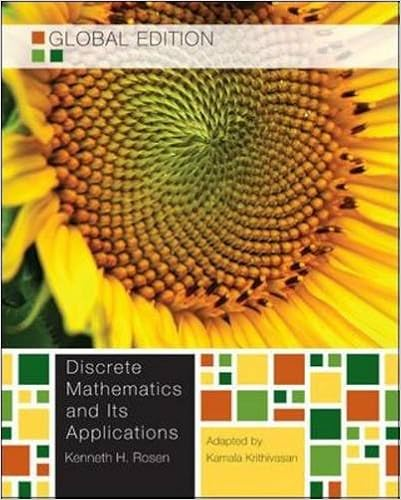semester details updated uploads MAT 258 Discrete Mathematics click headersin the left columnto expandor collapse This course gives an introduction to several mathematical topics of foundational importance in the mathematical and computer sciences. Typically starting with propositional and first-order logic, the course considers applications to methods of mathematical proof and reasoning. Further topics include basic set theory, number theory, enumeration, recurrence relations, mathematical induction, generating functions, and basic probability. Other topics may include graph theory, asymptotic analysis, and finite automata. Prerequisites: MAT 200 or MAT 230 Fall 2018 schedule WF 5:00-6:20PM in LECTURE THEATER 5B text Discrete Mathematics and Its Applications 7th Editionby Kenneth Rosen, Global Edition adapted by Kamala Krithivasandocuments course docs contains the syllabus and solutions to homework 1 through 7 slides sets and functions (tables and figures), number theory, relations (tables and figures), logic and proofs (tables and lists) contact mat258@mdvsamson.work please send me your available times when requesting a meeting office hours where and when I am in office announcements 6 november 2018 This is a reminder that the second examination will be given tomorrow, November 7, at Lecture Theater 5B, from 5:00pm to 6:30pm. The examination covers logic and proofs: logical equivalence proof methods knight-knave questions - unlike the previous questions which are metapuzzles, where some responses are not given, these are more straightforward The formula sheet is attached. There will be some questions involving divisibility and gcf. 11 october 2018 This is a reminder that the first examination will be given tomorrow, October 12, at Lecture Theater 5B, from 5:00pm to 6:30pm. The examination covers relations, and some topics leading up to relations: Chinese remainder theorem properties of relations on a set: reflexivity, symmetry, antisymmetry, transitivity representations of relations closures of relations equivalence relations and equivalence classes partial orders, total orders and topological sorts, Hasse diagrams, special elements of posets: minima/maxima, greatest/least elements There is no formula sheet. Bring a calculator if you will need it.